• 属于连续变量的有
千次阅读
2022-02-21 08:43:32

# 概念

相关性分析：两个连续变量之间的关系检验。

Pearson相关系数：衡量两个变量的线性相关关系；

Spearman相关系数：衡量两个变量的线性相关关系，部分非线性的也可以衡量；

Kendall相关系数：衡量两个变量之间非线性相关关系；

0.3以上就是有相关性了，0.3~0.5就是有点强的，0.5以上就是很强的。

下面是分类变量，研究非连续的变量

卡方检验：两个分类变量的分析，是否相关，不能表示强弱。

# Python例子

连续变量

代码如下：

from statsmodels.stats.anova import anova_lm
from statsmodels.formula.api import ols
import pandas as pd
from scipy import stats

df = pd.DataFrame(
[
[20, 6000], [18, 6500], [17, 4500], [16, 3000], [21, 8000], [23, 18000],
[30, 25000], [40, 18000], [55, 10000], [35, 19000], [26, 15000], [27, 8000]
],
columns=["age", "income"]
)

if __name__ == '__main__':

print(df[['age', 'income']].corr(method='pearson'), "\n")
print(df[['age', 'income']].corr(method='spearman'), "\n")
print(df[['age', 'income']].corr(method='kendall'), "\n")
print(stats.chi2_contingency(df));

pass

运行截图如下：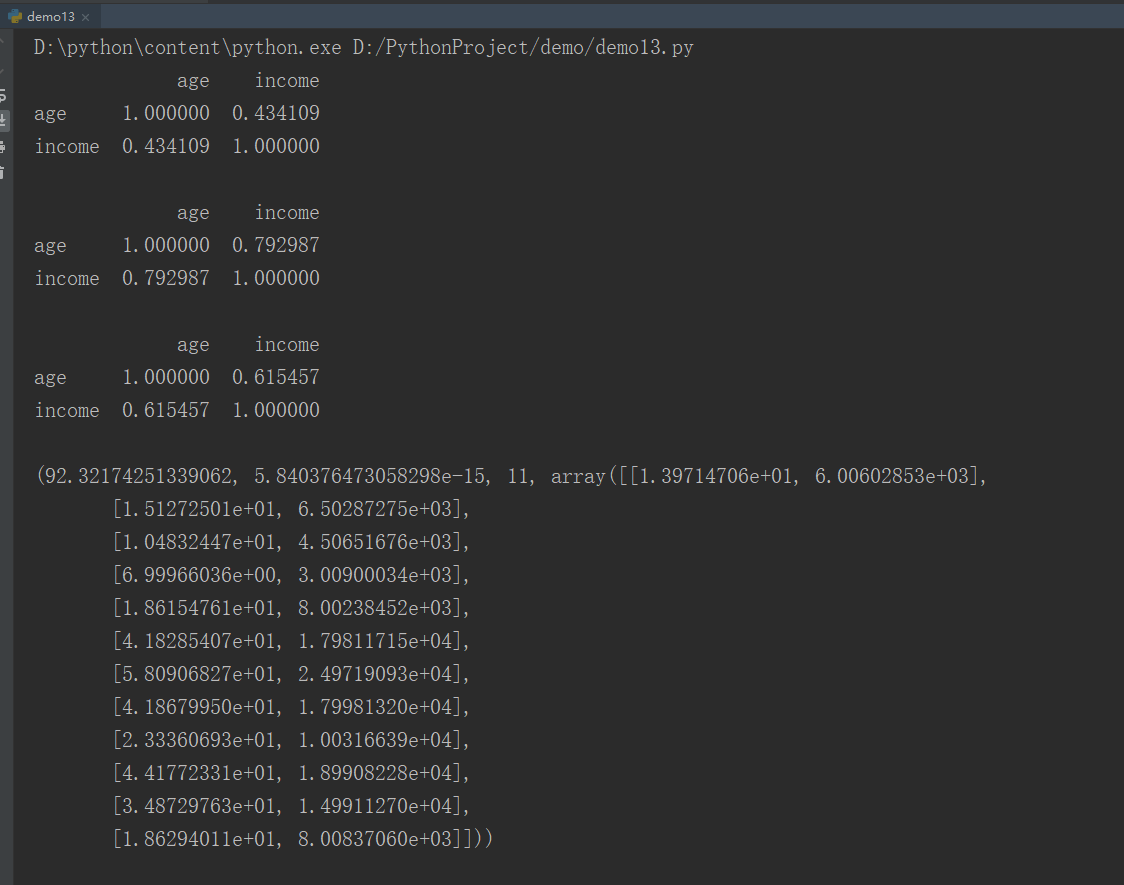python 分类 数据挖掘
更多相关内容
• 【060期】李克特量表的变量属于顺序变量，为什么可以当作连续变量使用？.docx
• ## 连续变量分箱

千次阅读 2020-11-20 09:27:28
文章目录1.变量分箱对模型的好处2....变量分箱方法主要用于连续变量，对于变量取值较稀疏的离散变量也应该进行分箱处理。 比如借款人的地址信息往往非常稀疏，通常先对地址信息处理到省或市，用每个省或市的坏样本

变量分箱主要是对连续变量离散化

对特征的一个优化过程

变量分箱（特征分箱）是一种特征工程方法，意在增强变量的可解释性与预测能力。变量分箱方法主要用于连续变量，对于变量取值较稀疏的离散变量也应该进行分箱处理。

比如借款人的地址信息往往非常稀疏，通常先对地址信息处理到省或市，用每个省或市的坏样本比率进行数值化，将数值化后的变量作为连续变量进行分箱.

# 1.变量分箱对模型的好处

• 1.降低异常值的影响，增加模型的稳定性
• 通过分箱来降低噪声，使模型鲁棒性更好
• 2.缺失值作为特殊变量参与分箱，减少缺失值填补的不确定性（分箱还可以解决缺失值 ）
• 通常的做法是，离散特征将缺失值转为字符串作为特殊字符即可，
• 而连续特征将缺失值作为特殊值即可
• 在后面的代码中连续值填充-777，离散值填充NA
• 3.增加变量的可解释性
• 分箱的方法往往要配合变量编码使用，这就大大提高了变量的可解释性
• 4.增加变量的非线性
• 提高了模型的拟合能力
• 5.增加模型的预测效果
• 通常假设训练集和测试集满足同分布，分箱使连续变量离散化，更容易满足同分布的假设
• 即减少模型在训练集的表现和测试集的偏差

# 2.分箱的局限

• 1.同一箱内的样本具有同质性
    分箱的基本假设是分在一个箱内的样本（借款人）具有相同
的风险等级，比如按年龄分箱的结果为{[18,25]，[25,40]，
[40,55]，[55,100]}，也就是将年龄在 18～25 的借款人统一按照
同一个数值变量来代替。对于树模型就减少了模型选择最优切分
点的可选择范围，会对模型的预测能力产生影响，损失了模型的
分辨能力

• 2.需要专家经验支持

# 3.变量分箱要注意的问题

分箱分的不好的话有些值的预测能力会忽略 会影响模型的预测能力 削弱模型的预测能力

• 1.分箱结果不宜过多
• 分箱过多导致特征过于稀疏，编码后的特征维度快速增加，使特征更加稀疏，会降低模型的预测效果
• 极端例子 一共100个样本 分了100个箱子 严重失衡
• 2.分箱结果不宜过少
• 每个箱子默认是同质的即风险等级相同
• 如果分箱过少则可能会造成模型辨识度过低
• 例如，年龄分箱结果为{[18,50]，[50,100]}，认为18～50岁的借款人风险水平相同这是不符合业务解释的。
• 3.分箱后单调性的要求

# 4.变量分箱的流程

变量分箱的目的是增加变量的预测能力或减少变量的自身冗余。

当预测能力不再提升或冗余性不再降低时，则分箱完毕。

因此，分箱过程是一个优化过程，所有满足上述要求的指标都可以用于变量分箱，这个指标也可叫作目标函数，可以终止或改变分箱的限制就是优化过程的约束条件# 5.卡方分箱

• 基本思想：

自底向上的分箱方法，相邻区间合并计算卡方值，卡方值越小说明两个区间的类分布越相似，合并两个区间

由设定的阈值决定（自由度、置信度），小于阈值就分箱

自底向上：由多至少逐层合并的过程

自顶向下是由少至多逐层切分的过程

数值特征离散化

特征之间强相关不好，但是某个特征和标签相关是好的

强相关：一个特征可以用另一个特征线性表示

• 解释性强
• 能解决多分类场景的分箱
• 缺点是计算量大
• 需要先对数值型变量离散化，然后迭代的计算卡方值

公式：上述过程就是一个卡方检验的过程，因此，根据置信度和自由度可以计算出卡方检验的阈值，当计算的卡方值小于阈值，则认为相邻区间的类分布情况相似，可进行合并。其中自由度为类别个数减 1，即本例中的自由度为 1；置信度可以使用 0.9、0.95和 0.99。

# 6.KS分箱

Best-KS 分箱方法是一种自顶向下的分箱方法。与卡方分箱相比，Best-KS分箱方法只是目标函数采用了 KS 统计量，其余分箱步骤没有差别

注意KS只能处理连续变量

可以用于模型对好坏样本的区分能力

• 基本思想：

根据KS曲线，取TPR和FPR之间的最大差值，就是KS统计率，也就是KS分箱最优切分点的位置

• KS曲线
• 横轴就是认为设定的阈值，就是区分好坏样本的界限
• 纵轴：一个是真正率TPR，一个是假正率FPR
• 之间的差值一定程度反映模型对好坏样本的区分能力
• 我们希望真正率高一点，假正率低一点（好样本多一点，坏样本少一点）
• 真正率：正样本预测数 / 正样本实际数
• TP /（TP + FN）
• 假正率：被预测为正的负样本结果数 / 负样本实际数
• FP /（FP + TN）

KS分箱过程也就是递归的找最优切分点的过程

KS值越大 模型的区分能力越强# 7.混淆矩阵概念复习• 召回率，真正率（recall）：TP/(TP+FN)
• 准确率（accuracy）：(TP+TN) / (TP+TN+FP+FN)
• 预测正确的 / 总样本数
• 精确率（precision）：TP / (TP+FP)
• 预测为1且正确 / 所有预测为1的样本数

# 8.最优IV分箱

最优 IV 分箱方法也是自顶向下的分箱方式，其目标函数为 IV 值

IV 值其本质是对称化的 K-L 距离，即在切分点处分裂得到的两部分数据中，选择好坏样本的分布差异最大点作为最优切分点。分箱结束后，计算每个箱内的 IV 值加和得到变量的 IV 值，可以用来刻画变量对目标值的预测能力。即变量的 IV 值越大，则对目标变量的区分能力越强，因此，IV 值还可以用来做变量选择。

# 9.基于树的最优分箱方法

基于树的分箱方法借鉴了决策树在树生成的过程中特征选择(最优分裂点)的目标函数来完成变量分箱过程，可以理解为单变量的决策树模型。决策树采用自顶向下递归的方法进行树的生成，每个节点的选择目标是为了分类结果的纯度更高，也就是样本的分类效果更好。因此，不同的损失函数有不同的决策树，ID3采用信息增益方法，C4.5 采用信息增益比，CART 采用基尼系数（Gini）指标

# 10.分箱框架源码（卡方、最优IV、信息增益）

# -*- coding: utf-8 -*-
import os
import pandas as pd
import numpy as np
import pickle
from sklearn.preprocessing import OneHotEncoder
from sklearn.preprocessing import LabelEncoder
from sklearn.model_selection import train_test_split
import warnings

warnings.filterwarnings("ignore")  ##忽略警告

# 注意sklearn版本要在v.20.0以上，不同版本函数的位置会不同。
df = pd.read_csv(os.path.join(data_path, file_name), delim_whitespace=True, header=None, engine='python')
# 变量重命名
columns = ['status_account', 'duration', 'credit_history', 'purpose', 'amount',
'svaing_account', 'present_emp', 'income_rate', 'personal_status',
'other_debtors', 'residence_info', 'property', 'age',
'inst_plans', 'housing', 'num_credits',
'job', 'dependents', 'telephone', 'foreign_worker', 'target']
df.columns = columns

# 将标签变量由状态1,2转为0,1;0表示好用户，1表示坏用户
df.target = df.target - 1

# 数据分为data_train和 data_test两部分，训练集用于得到编码函数，验证集用已知的编码规则对验证集编码
# x_train, x_test, y_train, y_test = train_test_split(x, y, test_size=0.2)
# stratify： 依据标签y，按原数据y中各类比例，分配给train和test，使得train和test中各类数据的比例与原数据集一样
data_train, data_test = train_test_split(df, test_size=0.2, random_state=0, stratify=df.target)
return data_train, data_test

# one—hot编码
# df: 数据框  data_path_1：编码模型保存的位置  flag：数据集
def onehot_encode(df, data_path_1, flag='train'):
# reset_index：重置索引， drop=True：不想保留原来的index
df = df.reset_index(drop=True)

# 判断数据集是否存在缺失值  如果是进行缺失值填补
# df.isnull().any() 判断哪些列存在缺失值
if sum(df.isnull().any()) > 0:
# 数值型和字符串型特征拿出来
numerics = ['int16', 'int32', 'int64', 'float16', 'float32', 'float64']
var_numerics = df.select_dtypes(include=numerics).columns
var_str = [i for i in df.columns if i not in var_numerics]

# 数据类型的缺失值用-77777填补
if len(var_numerics) > 0:
df.loc[:, var_numerics] = df[var_numerics].fillna(-7777)

# 字符串类型的缺失值用NA填补
if len(var_str) > 0:
df.loc[:, var_str] = df[var_str].fillna('NA')

# pickle.dump(obj, file, [,protocol])  序列化对象，将对象obj保存到文件file中去
# 参数protocol是序列化模式，默认是0（ASCII协议，表示以文本的形式进行序列化）
if flag == 'train':
enc = OneHotEncoder(dtype='int').fit(df)
# 保存编码模型
with open(os.path.join(data_path_1, 'onehot.pkl'), 'wb') as save_model:
pickle.dump(enc, save_model, 0)

df_return = pd.DataFrame(enc.transform(df).toarray())
df_return.columns = enc.get_feature_names(df.columns)

elif flag == 'test':
# 测试数据编码
with open(os.path.join(data_path_1, 'onehot.pkl'), 'rb') as read_model:

# 如果训练集无缺失值，测试集有缺失值则将该样本删除
var_range = onehot_model.categories_  # 训练集one-hot编码后的类别种类
var_name = df.columns
del_index = []
for i in range(len(var_range)):
if 'NA' not in var_range[i] and 'NA' in df[var_name[i]].unique():
index = np.where(df[var_name[i]] == 'NA')
del_index.append(index)
elif -7777 not in var_range[i] and -7777 in df[var_name[i]].unique():
index = np.where(df[var_name[i]] == -7777)
del_index.append(index)

# 删除样本
if len(del_index) > 0:
del_index = np.unique(del_index)
df = df.drop(del_index)
print('训练集无缺失值，但测试集有缺失值，第{0}条样本被删除'.format(del_index))
df_return = pd.DataFrame(onehot_model.transform(df).toarray())
df_return.columns = onehot_model.get_feature_names(df.columns)

elif flag == 'transform':
# 编码数据值转化为原始变量
with open(os.path.join(data_path_1, 'onehot.pkl'), 'rb') as read_model:

# 逆变换
df_return = pd.DataFrame(onehot_model.inverse_transform(df))
df_return.columns = np.unique(['_'.join(i.rsplit('_')[:-1]) for i in df.columns])

return df_return

# 标签编码
def label_encode(df, data_path_1, flag='train'):
if flag == 'train':
enc = LabelEncoder().fit(df)
# 保存编码模型
with open(os.path.join(data_path_1, 'labelcode.pkl'), 'wb') as save_model:
pickle.dump(enc, save_model, 0)

df_return = pd.DataFrame(enc.transform(df))
df_return.name = df.name

elif flag == 'test':
# 测试数据编码
with open(os.path.join(data_path_1, 'labelcode.pkl'), 'rb') as read_model:

df_return = pd.DataFrame(label_model.transform(df))
df_return.name = df.name

elif flag == 'transform':
# 编码数据值转化为原始变量
with open(os.path.join(data_path_1, 'labelcode.pkl'), 'rb') as read_model:

# 逆变换
df_return = pd.DataFrame(label_model.inverse_transform(df))
return df_return

# 自定义映射
def dict_encode(df, data_path_1):
# 自定义映射
embarked_mapping = {}
embarked_mapping['status_account'] = {'NA': 1, 'A14': 2, 'A11': 3, 'A12': 4, 'A13': 5}
embarked_mapping['svaing_account'] = {'NA': 1, 'A65': 1, 'A61': 3, 'A62': 5, 'A63': 6, 'A64': 8}
embarked_mapping['present_emp'] = {'NA': 1, 'A71': 2, 'A72': 5, 'A73': 6, 'A74': 8, 'A75': 10}
embarked_mapping['property'] = {'NA': 1, 'A124': 1, 'A123': 4, 'A122': 6, 'A121': 9}

df = df.reset_index(drop=True)

# 判断数据集是否存在缺失值
if sum(df.isnull().any()) > 0:
df = df.fillna('NA')

# 字典映射
var_dictEncode = []
for i in df.columns:
col = i + '_dictEncode'
df[col] = df[i].map(embarked_mapping[i])
var_dictEncode.append(col)
return df[var_dictEncode]

# WOE编码
# 返回某个特征的woe映射后的df、woe字典、iv值
# x:特征   y:类别   target：正样本为1
def woe_cal_trans(x, y, target=1):
# 计算总体的正负样本数
p_total = sum(y == target)  # 正样本数
n_total = len(x) - p_total  # 负样本数
value_num = list(x.unique())  # 去重后的总数
woe_map = {}
iv_value = 0
for i in value_num:  # 这个特征每种取值的woe值
# 计算该变量取值箱内的正负样本总数
y1 = y[np.where(x == i)]
p_num_1 = sum(y1 == target)
n_num_1 = len(y1) - p_num_1
# 计算占比
# bad_1 = p_num_1 / p_total  # 坏样本分布率
# good_1 = n_num_1 / n_total  # 好样本分布率
good_1 = p_num_1 / p_total  # 坏样本分布率
bad_1 = n_num_1 / n_total  # 好样本分布率
if bad_1 == 0:
elif good_1 == 0:
good_1 = 1e-5
woe_map[i] = np.log(bad_1 / good_1)  # woe值
iv_value += (bad_1 - good_1) * woe_map[i]  # iv值
x_woe_trans = x.map(woe_map)
x_woe_trans.name = x.name + "_woe"

return x_woe_trans, woe_map, iv_value

# WOE编码映射
def woe_encode(df, data_path_1, varnames, y, filename, flag='train'):
"""
Param:
df: 待编码数据
data_path_1 :存取文件路径
varnames: 变量列表
y:  目标变量
filename:编码存取的文件名
flag: 选择训练还是测试
---------------------------------------
Return:
df: 编码后的数据，包含了原始数据
woe_maps: 编码字典
iv_values: 每个变量的IV值
var_woe_name: 每个特征拼接woe的列名
"""
df = df.reset_index(drop=True)  # 重置索引，不保留原来的索引

# 判断数据集是否存在缺失值
if sum(df.isnull().any()) > 0:
numerics = ['int16', 'int32', 'int64', 'float16', 'float32', 'float64']
var_numerics = df.select_dtypes(include=numerics).columns  # 数值型特征
var_str = [i for i in df.columns if i not in var_numerics]  # 字符串型特征
# 数据类型的缺失值用-77777填补
if len(var_numerics) > 0:
df.loc[:, var_numerics] = df[var_numerics].fillna(-7777)
# 字符串类型的缺失值用NA填补
if len(var_str) > 0:
df.loc[:, var_str] = df[var_str].fillna('NA')

if flag == 'train':
iv_values = {}  # 保存每个特征的iv值
woe_maps = {}  # 保存每个特征的woe值
var_woe_name = []
for var in varnames:  # 遍历每一个特征
x = df[var]
# 变量映射
x_woe_trans, woe_map, info_value = woe_cal_trans(x, y)
var_woe_name.append(x_woe_trans.name)
df = pd.concat([df, x_woe_trans], axis=1)  # 按行拼接woe值
woe_maps[var] = woe_map
iv_values[var] = info_value

# 保存woe映射字典
with open(os.path.join(data_path_1, filename + '.pkl'), 'wb') as save_woe_dict:
pickle.dump(woe_maps, save_woe_dict, 0)

return df, woe_maps, iv_values, var_woe_name

elif flag == 'test':
# 测试数据编码
with open(os.path.join(data_path_1, filename + '.pkl'), 'rb') as read_woe_dict:

# 如果训练集无缺失值，测试集有缺失值则将该样本删除
woe_dict.keys()
del_index = []
for key, value in woe_dict.items():
if 'NA' not in value.keys() and 'NA' in df[key].unique():
index = np.where(df[key] == 'NA')
del_index.append(index)
elif -7777 not in value.keys() and -7777 in df[key].unique():
index = np.where(df[key] == -7777)
del_index.append(index)
# 删除样本
if len(del_index) > 0:
del_index = np.unique(del_index)
df = df.drop(del_index)
print('训练集无缺失值，但测试集有缺失值，该样本{0}删除'.format(del_index))

# WOE编码映射
var_woe_name = []
for key, value in woe_dict.items():
val_name = key + "_woe"
df[val_name] = df[key].map(value)
var_woe_name.append(val_name)

return df, var_woe_name

if __name__ == '__main__':
path = r'G:\A1\python_workspace\finance_code\chapter5\\'
data_path = os.path.join(path, 'data')
file_name = 'german.csv'
# 读取数据
data_train, data_test = data_read(data_path, file_name)
# 不可排序变量
var_no_order = ['credit_history', 'purpose', 'personal_status', 'other_debtors',
'inst_plans', 'housing', 'job', 'telephone', 'foreign_worker']

# x_woe_trans, woe_map, iv_value = woe_cal_trans(data_train['job'], data_test['target'])
# print(x_woe_trans)
# print(woe_map)
# print(iv_value)

# one-hot编码
# 训练数据编码
data_train.credit_history = np.nan
data_train_encode = onehot_encode(data_train[var_no_order], data_path, flag='train')

# 测试集数据编码
data_test.credit_history = np.nan
data_test.purpose = np.nan
data_test_encode = onehot_encode(data_test[var_no_order], data_path, flag='test')

# 查看编码逆变化后的原始变量名
df_encoded = data_test_encode.loc[0:4]
data_inverse = onehot_encode(df_encoded, data_path, flag='transform')
print(data_inverse)

# 哑变量编码
data_train_dummies = pd.get_dummies(data_train[var_no_order])
data_test_dummies = pd.get_dummies(data_test[var_no_order])
print(data_train_dummies.columns)

# 可排序变量
# 注意，如果分类变量的标签为字符串，这是需要将字符串数值化才可以进行模型训练，标签编码其本质是为
# 标签变量数值化而提出的方法，因此，其值支持单列数据的转化操作，并且转化后的结果是无序的。
# 因此有序变量统一用字典映射的方式完成。
var_order = ['status_account', 'svaing_account', 'present_emp', 'property']

# 标签编码
# 训练数据编码
data_train_encode = label_encode(data_train[var_order], data_path, flag='train')

# 验证集数据编码
data_test_encode = label_encode(data_test[var_order], data_path, flag='test')

# 查看编码你变化后的原始变量名
# 后面再改一下
df_encoded = data_test_encode
data_inverse = label_encode(df_encoded, data_path, flag='transform')

# 自定义映射
# 训练数据编码
data_train.credit_history = np.nan
data_train_encode = dict_encode(data_train[var_order], data_path)

# 测试集数据编码
data_test.status_account = np.nan
data_test_encode = dict_encode(data_test[var_order], data_path)
print(data_test_encode)

# WOE编码
# 训练集WOE编码
df_train_woe, dict_woe_map, dict_iv_values, var_woe_name = woe_encode(data_train, data_path, var_no_order,
data_train.target, 'dict_woe_map',
flag='train')
print(df_train_woe, '\n')
print(dict_woe_map, '\n')
print(dict_iv_values, '\n')
print(var_woe_name, '\n')

# 测试集WOE编码
df_test_woe, var_woe_name = woe_encode(data_test, data_path, var_no_order, data_train.target, 'dict_woe_map',
flag='test')

print(df_train_woe)


展开全文机器学习
• 1李克特量表的变量属于顺序变量，为什么可以当作连续变量使用？ 1.1导读 心理学和社会科学使用的问卷或量表基本上很难达到连续变量的水平，如李克特量表很难取小数（尽管将其视为连续变量对待），因此，社会科学研究...

## 一、教学内容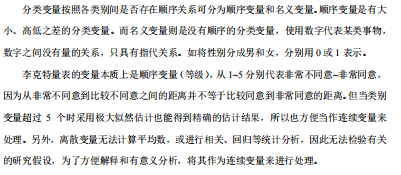## 二、备注

相关资料已上传我的资源，下载链接https://blog.csdn.net/TIQCmatlab?spm=1011.2124.3001.5343

展开全文• 因此我们需要将年龄分组，比如0-20岁、20-30岁等，此时就要将年龄转换为类别变量。 group 等分数据 必须先对数据进行排序 gen g_pri = group(price) recode 指定分界点 前开后闭区间 recode price (min/5000 ...

在数据分析过程中，我们通常关心一个区间而非具体某个点的情况，如年龄对工资的影响，显然35岁与36岁没有本质区别。因此我们需要将年龄分组，比如0-20岁、20-30岁等，此时就要将年龄转换为类别变量。

group

• 等分数据
• 必须先对数据进行排序
gen g_pri = group(price)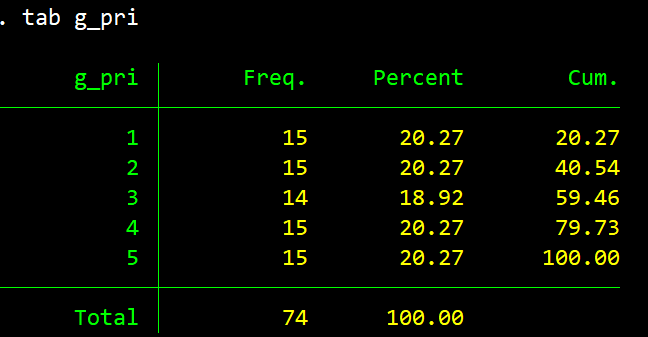recode

• 指定分界点
• 前开后闭区间
 recode price (min/5000 = 1) (5000/8000 = 2) (8000/max = 3), gen(g_price)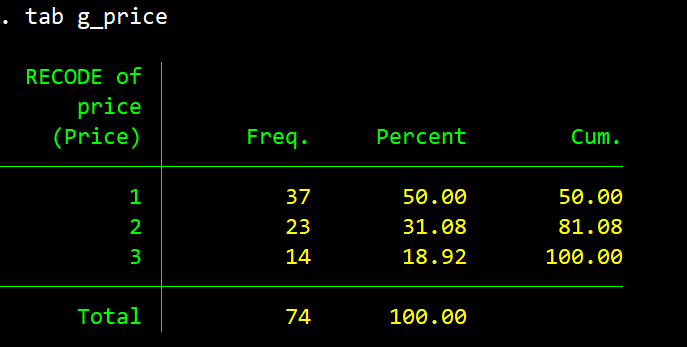irecode

• 函数，同recode
• 命令更简洁
gen ig_pri = irecode(price, 5000, 8000)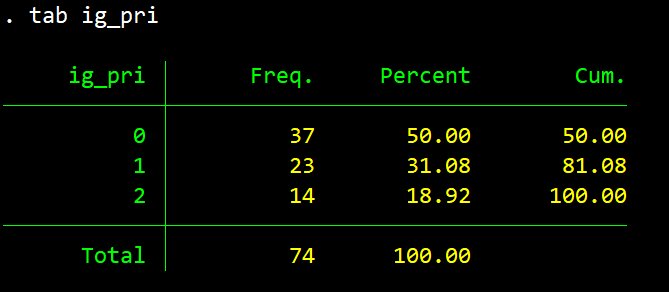cond

• 条件函数，和三目运算差不多
• 语法：cond(x, a, b)，满足x返回a否则返回b
• 将数据分为两组时可用
gen c_price = cond(price > 8000, 1, 0)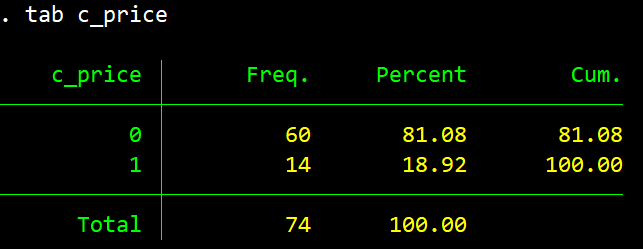展开全文• Meta分析简明教程：No.30 连续变量的STATA操作.pptx
• 反之,在一定区间内可以任意取值的变量叫连续变量,其数值是连续不断的,相邻两个数值可作无限分割,即可取无限个数值.例如,生产零件的规格尺寸,人体测量的身高,体重,胸围等为连续变量,其数值只能用测量或计量的方法取得...
• 优化 | 线性化：0-1变量乘以连续变量的线性化非线性整数规划模型Gurobi求解代码线性化总结 作者：刘兴禄， 清华大学 清华-伯克利深圳学院，博士在读 欢迎关注我们的微信公众号 运小筹 非线性整数规划模型 考虑下面...
• Python变量重分类/Python变量重编码（连续变量重分类、离散变量重分类）python 分类
• 定量变量可以分为离散变量和连续变量。 类别变量 类别变量包含有限的类别数或可区分组数。类别数据可能不是逻辑顺序。例如，类别变量包括性别、材料类型和付款方式。 离散变量 离散变量是在任意两个值之间具有可...数据结构
• 连续变量的统计描述与参数估计1 连续变量的统计描述1.1 集中趋势的描述指标1.2 离散趋势的描述指标1.3 正态分布的描述指标2 连续变量的参数估计2.1 正态分布2.2 参数的点估计2.3 参数的区间估计3 Bootstrap方法 ...
• 在表格数据集上创建任何机器学习模型之前, 通常我们会检查独立变量和...在本文中, 我们将了解如何找到分类变量和连续变量之间的相关性。案例 1: 当独立变量只有两个值时点双性关联如果分类变量只有两个值 (即 true...
• 机器学习 决策树篇——解决连续变量的分类问题摘要信息熵、条件熵、熵增益、熵增益率的计算GiNi系数、GiNi系数增益的计算python代码连续变量决策树分类案例 摘要 本文通过python实现了连续变量的信息熵、条件熵、熵...python 决策树 机器学习 数据挖掘
• 第一个问题，连续变量是什么？ 一个人的年级，统计出来1、2、3…… 一个人的年纪，统计出来18、19、20…… 但是，虽然都是数字，只有年纪是连续变量。 为什么？顾名思义，年纪是连续的，18 - 19之间可以无限划分...
• 戳戳原链接：...分类变量（categorical var...
• 我们需要对不同类型字段进行转化。并且在此过程中，我们需要检验是否存在采用别的值 来表示缺失值的情况。就像此前所说我们通过isnull只能检验出None(Python原生对象...需要注意的是，如果是连续变量，则无法使用上述方python
• 在日常的数据分析工作中，经常需要把数据变量转换成模型需要的样子，比如我们经常遇见的数据都是标签化、文字化等内容，需要将这些数据转换成计算机看得懂的内容，就是需要进行标签变量的转换。不管是离散数据还是...python 机器学习
• 数据离散化是指将连续的数据进行分段，使其变为一段段离散化的区间。分段的原则基于等距离、等频率或优化的方法。数据离散化的原因主要以下几点： 算法需要 比如决策树、朴素贝叶斯等算法，都是基于离散型的...数据挖掘 机器学习
• 单臂连续变量（直接合并效应量）Meta分析，连续变量
• 连续变量离散化三种方法 1.等宽离散化 2.等频离散化 3.利用聚类进行离散化 import numpy as np import pandas as pd #参数初始化 datafile = './data/discretization_data.xls' #读取数据 data = pd.read_...
• 反之,在一定区间内可以任意取值的变量叫连续变量,其数值是连续不断的,相邻两个数值可作无限分割,即可取无限个数值.例如,生产零件的规格尺寸,人体测量的身高,体重,胸围等为连续变量,其数值只能用测量或计量的方法取得...连续型和离散型
• 在表格数据集上创建任何机器学习模型之前, 通常我们会检查独立变量和...在本文中, 我们将了解如何找到分类变量和连续变量之间的相关性。案例 1: 当独立变量只有两个值时点双性关联如果分类变量只有两个值 (即 true...
• 周岁年龄”这个变量进行连续变量的频率分析： 步骤：【分析】-【描述统计】-【频率】-【Q3.周岁年龄】 在【频率：统计】中，SPSS提供了丰富的描述性计量，包括百分位值、集中趋势、离散趋势和数据分布特征4个部分...大数据
• R语言连续变量等级划分 ###################等级划分################### ###数据读取 dat <- read.csv("F:/Desktop/ccv.csv") # ##查看数据结构 # str(dat) ##########以按HT(优势树高)划分等级为例######...
• 原文链接：http://tecdat.cn/?p=18169 比如说分类变量为是否幸存、是因变量，连续变量为年龄、是自变量，这两者可以做相关分析吗？两者又是否可以做回归分析？ 我们考虑泰坦尼克号数据集，
• 遇到一个二进制变量与一个连续变量相乘的形式，可以通过大M算法来线性化，转化后的形式也可以被cvx接受。 大M算法见以下链接： ... ...
• SPSS如何做离散和连续变量的统计描述 离散变量的统计描述 原始数据 §频数列表 §百分比 §累计频数 §累计百分比 集中趋势 §众数 连续变量的统计描述 频数表 操作步骤：确定组数；确定组距；确定各组...
• 连续变量的统计描述指标体系 集中趋势（位置统计量）：均数适用于正态分布和对称分布资料；中位数适用于所有分布资料 离散趋势（尺度统计量）：标准差、方差只适用于正态分布资料；四分位数适用于各种分布...
• Excel 文件转成stata文件工具： Stata Transfer 一、中介效应(Mediating effects) 仔细展示一下代码 自变量 council_pre 理事长 council_se 秘书长 逐步检验回归系数 //检验方程一 reg 因变量变量 控制变量 //......